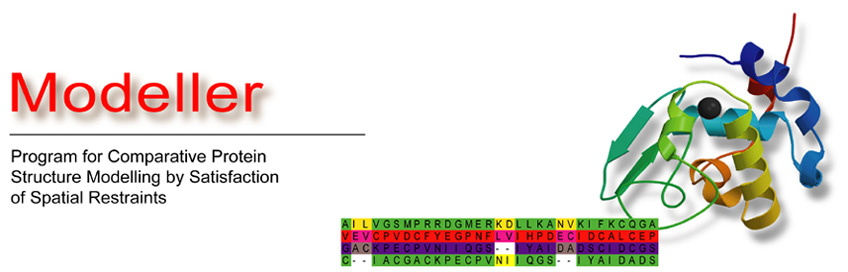• Diff for "Mutate model"
Differences between revisions 9 and 10
 ⇤ ← Revision 9 as of 2008-06-04 01:51:44 → Size: 5590 Editor: ModellerCaretaker Comment: page was renamed from Mutate_model ← Revision 10 as of 2008-07-09 01:53:42 → ⇥ Size: 5946 Editor: ModellerCaretaker Comment: Add note about changing rand_seed to change models. Deletions are marked like this. Additions are marked like this. Line 1: Line 1: ## page was renamed from Mutate_model Line 3: Line 2: Note that this script if run multiple times will produce the same model each time, because Modeller is deterministic. If you want to build multiple models, change the value of `rand_seed` (see comments in the script) each time. This may be useful if some models, for example, cannot be optimized due to steric clashes. Line 71: Line 72: # Set a different value for rand_seed to get a different final model Line 72: Line 74: Line 75: Line 78: #lennard-jones potential (more accuate) #lennard-jones potential (more accurate)

The script below takes a given PDB file, and mutates a single residue. The residue's position is then optimized, and the unoptimized and optimized energies are reported.

Note that this script if run multiple times will produce the same model each time, because Modeller is deterministic. If you want to build multiple models, change the value of rand_seed (see comments in the script) each time. This may be useful if some models, for example, cannot be optimized due to steric clashes.

import sys
import os

from modeller import *
from modeller.automodel import autosched

#
#  mutate_model.py
#
#     Usage:   mod9v2 - modelname respos resname chain < mutate_model.py > logfile
#
#     Example: mod9v2 - 1t29 1699 LEU A < mutate_model.py > 1t29.log
#
#
#  Creates a single in silico point mutation to sidechain type and at residue position
#  input by the user, in the structure whose file is modelname.pdb
#  The conformation of the mutant sidechain is optimized by conjugate gradient and
#  refined using some MD.
#

def optimize(atmsel, sched):
for step in sched:
step.optimize(atmsel, max_iterations=200, min_atom_shift=0.001)
#md
refine(atmsel)
cg.optimize(atmsel, max_iterations=200, min_atom_shift=0.001)

#molecular dynamics
def refine(atmsel):
# at T=1000, max_atom_shift for 4fs is cca 0.15 A.
md = molecular_dynamics(cap_atom_shift=0.39, md_time_step=4.0,
md_return='FINAL')
init_vel = True
for (its, equil, temps) in ((200, 20, (150.0, 250.0, 400.0, 700.0, 1000.0)),
(200, 600,
(1000.0, 800.0, 600.0, 500.0, 400.0, 300.0))):
for temp in temps:
md.optimize(atmsel, init_velocities=init_vel, temperature=temp,
max_iterations=its, equilibrate=equil)
init_vel = False

#use homologs and dihedral library for dihedral angle restraints
def make_restraints(mdl1, aln):
rsr = mdl1.restraints
rsr.clear()
s = selection(mdl1)
for typ in ('stereo', 'phi-psi_binormal'):
rsr.make(s, restraint_type=typ, aln=aln, spline_on_site=True)
for typ in ('omega', 'chi1', 'chi2', 'chi3', 'chi4'):
rsr.make(s, restraint_type=typ+'_dihedral', spline_range=4.0,
spline_dx=0.3, spline_min_points = 5, aln=aln,
spline_on_site=True)

#first argument
modelname, respos, restyp, chain, = sys.argv[1:]

log.verbose()

# Set a different value for rand_seed to get a different final model
env = environ(rand_seed=-49837)

env.io.hetatm = True
#soft sphere potential
env.edat.dynamic_sphere=False
#lennard-jones potential (more accurate)
env.edat.dynamic_lennard=True
env.edat.contact_shell = 4.0
env.edat.update_dynamic = 0.39

# Read customized topology file with phosphoserines (or standard one)

# Read customized CHARMM parameter library with phosphoserines (or standard one)

# Read the original PDB file and copy its sequence to the alignment array:
mdl1 = model(env, file=modelname)
ali = alignment(env)
ali.append_model(mdl1, atom_files=modelname, align_codes=modelname)

#set up the mutate residue selection segment
s = selection(mdl1.chains[chain].residues[respos])

#perform the mutate residue operation
s.mutate(residue_type=restyp)
#get two copies of the sequence.  A modeller trick to get things set up
ali.append_model(mdl1, align_codes=modelname)

# Generate molecular topology for mutant
mdl1.clear_topology()
mdl1.generate_topology(ali[-1])

# Transfer all the coordinates you can from the template native structure
# to the mutant (this works even if the order of atoms in the native PDB
# file is not standard):
#here we are generating the model by reading the template coordinates
mdl1.transfer_xyz(ali)

# Build the remaining unknown coordinates
mdl1.build(initialize_xyz=False, build_method='INTERNAL_COORDINATES')

#yes model2 is the same file as model1.  It's a modeller trick.
mdl2 = model(env, file=modelname)

#required to do a transfer_res_numb
#ali.append_model(mdl2, atom_files=modelname, align_codes=modelname)
#transfers from "model 2" to "model 1"
mdl1.res_num_from(mdl2,ali)

#It is usually necessary to write the mutated sequence out and read it in
#before proceeding, because not all sequence related information about MODEL
#is changed by this command (e.g., internal coordinates, charges, and atom
#types and radii are not updated).

mdl1.write(file=modelname+restyp+respos+'.tmp')

#set up restraints before computing energy
#we do this a second time because the model has been written out and read in,
#clearing the previously set restraints
make_restraints(mdl1, ali)

#a non-bonded pair has to have at least as many selected atoms
mdl1.env.edat.nonbonded_sel_atoms=1

sched = autosched.loop.make_for_model(mdl1)

#only optimize the selected residue (in first pass, just atoms in selected
#residue, in second pass, include nonbonded neighboring atoms)
#set up the mutate residue selection segment
s = selection(mdl1.chains[chain].residues[respos])

mdl1.restraints.unpick_all()
mdl1.restraints.pick(s)

s.energy()

s.randomize_xyz(deviation=4.0)

mdl1.env.edat.nonbonded_sel_atoms=2
optimize(s, sched)

#feels environment (energy computed on pairs that have at least one member
#in the selected)
mdl1.env.edat.nonbonded_sel_atoms=1
optimize(s, sched)

s.energy()

#give a proper name
mdl1.write(file=modelname+restyp+respos+'.pdb')

#delete the temporary file
os.remove(modelname+restyp+respos+'.tmp')